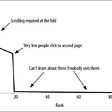# Collaborative Filtering Recommendation System — an Introduction

Just a kind note: Since Medium does not support maths formula, many of them in this post were changed to raw representation. In case you dislike reading them (as I), you can find better content here.

# Introduction

I introduced Content-based Recommendation Systems in the last article, which aims to provide item recommendations to the users based on the item’s features. Basically, the content-based approach builds a model for every user interest regardless of the presence of other users but items’ profiles. The advantage of this approach lies in its requirement of memory resources and computation time. It leverages the features of each item provided in the item’s description or specification which in fact can be defined in advance by the providers or collected via asking users to tag them. The construction of vector features for items is typically done by adopting the techniques like Natural Language Processing — NLP.

Nevertheless, there are two limits to the content-based approach. First, it does not take advantage of the information from other users when building the user’s interest model. Such information is useful considering the fact that users' behaviors can be classified into several simple groups and therefore if the behaviors of some members are known prior, the system should be able to deduce the behaviors of the rest. Second, the item’s specification is not always available or complete, i.e. very old songs or movies might lack some information or are given the wrong data. Asking users to tag them is even more challenging not only because not everyone would be willing to do so but also because it could be biased by their own personality. For the NLP algorithm, it requires more effort in handling special cases like typos, acronyms, or in different languages.

All of these issues can be tackled by Collaborative Filtering (CF) which is the main focus of this post. I will present to you a method called Neighborhood-based Collaborative Filtering (NBCF). In my next post, I will explain another one, called Matrix Factorization Collaborative Filtering. Having said that, and for the convenience, from now on, I mean CF by NBCF by default in this post.

`The idea of NBCF is to determine the interest of an user on an item based on other users that share some common characteristics with the user. Via this closeness among users, the system can measure how much the users are interested at items it has. For instance, user A, B are big fans of the series Breaking Bad (who does not? :)), as well as the fact user A also rates 5 stars for Prison Break. It is likely that user B can be also a big fan of the Prison Break.`

It is understandable two most important questions that an NBCF system needs to answeres:

• How to represent (and determine) the similarity between two users?
• If it could identify similar users, then how to predict the interest of a user on an item?

The method of determining the interest of a user on an item based on the interest of similar users on that item is called user-user collaborative filtering. Another method is item-item collaborative filtering that takes item similarities into account rather than user similarities and then recommends the items that are close to those highly interested by the user.

In the next sections, I will start with user-user collaborative filtering and its limitations. Then I will explain how those limitations can be addressed by item-item collaborative filtering. The discussion will be justified via examples based on the dataset MovieLens 100k that will be mentioned in my next article.

# User-user Collaborative Filtering

## Similarity functions

The most important task that needs to be done by user-user CF is to measure the similarity between two users. Given utility matrix Y as the only input, the similarity can be determined based on the columns corresponding with these two users. Let’s consider the following figure: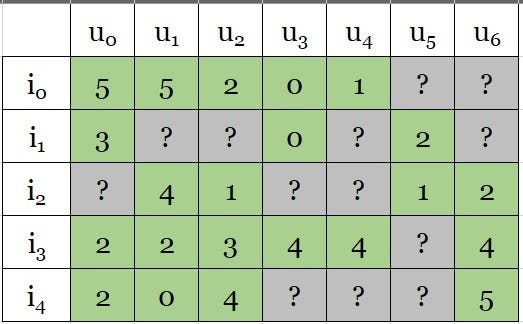Figure 1: Example of utility matrix based on the number of stars rated for an item. Intuitively, user u_0 behaves quite closer to user u_1 than to u_2, u_3, u_4, u_5, u_6. As u_1 is interested in item i_2, we can hope that \$u_0\$ is also interested in i_2.

Given users from u_0 to u_6, items from i_0 to i_4 and the matrix cells represent the number of stars rated by each user on each item. The greater the number is, the more a user shows interest in an item. The ? cells are what the system is looking for. We define the function sim(u_i, u_j) as the similarity between two users u_i and u_j.

At a glance, we can see that u_0 and u_1 like i_0, i_1, i_2 and do not like i_3, i_4 that much. This is in contrast to other users. Therefore, a good similarity function should satisfy the following condition:

From there, to determine the interest of user u_0 in the item i_2, we take the user u_1 into account and his interest in the item i_2. As u_1 likes \$i_2\$, the system should recommend i_2 to the user i_0.

The question is how to define or choose a good similarity function? The common approach of measuring the similarity between two users is based on the feature vector of each user and apply a certain function to calculate the similarity between these two vectors. Note that, unlike constructing item profiles in the Content-based approach, i.e. based on pre-defined description or specification or asking users to tag, the user’s feature vector is constructed from the utility matrix whose column for the user is the rating that he does on items, also the only data we have. What matters is the fact that these columns are quite sparse, i.e. with many incomplete “?” matrix elements since the user rates only very few items.

In order to mitigate this issue, we need to assist the system with initial raw values and of course, these raw values should not affect much the similarity between 2 vectors. Note that the initialization is only for calculating the similarity, and should not be used as the final output for the recommendation task. But which value should be used to replace “?”? There are a couple of options:

• Zero: This is not really a good idea when saying that a user who has not yet rated an item does not have any interest in that item.
• 2.5: This is the average between 0, not interested at all, and 5 truly interested, and seems to be better than 0. However, it is not an ideal option for extreme users who can rate 3 stars for non-interesting items by easy-to-go users or for interesting ones for tough persons.
• Mean ratings: This is the mean value of all the ratings done by the users on items. Doing this can lessen the bias in the case of extreme users. This idea is explained in Figure 2.

At the last line, the high value corresponds to the tough users and vice versa. By subtracting the mean value from the ratings and replacing “?” with 0, we obtain a normalized utility matrix as shown in Figure 3:

• Subtracting the mean value results in a column with both positive and negative values thanks to which the system can conclude that the user shows more interest in some items than the others. Zero items indicate that they have not yet received any interest from the user.
• Technically, the utility matrix is a high dimensional matrix with millions of users and items and requires a large memory resource to store all of its values. As there are quite a small number of known ratings compared to the utility matrix’s dimension, it is more efficient to use a data structure to represent a sparse matrix, i.e. with non-zero (and non-”?”) values and their location (row and column indices). By doing so, not only memory resource is optimized but also the performance of calculating the similarity matrix is improved. Here the element at i-th row and j-th column of the similarity matrix represents the similarity between user u_i and user u_j.

After normalizing the data, the following cosine function is used:

where u_1, u_2 are the vectors of user 1 and user 2 respectively. The similarity of two vectors is real in the range [-1, 1] in which 1 means the angle between these vectors is 0 and therefore implies two totally similar vectors. And -1 indicates two vectors with opposite directions and can be intuitively explained by the fact that two users with opposite behaviors have the lowest similarity.

Figure 4 demonstrates the cosine-based similarity matrix S between users given the normalized data in Figure 3. Since cosine is a symmetric function, the matrix S is a symmetric matrix and thus user A is similar to user B, then user B is also similar to user A. Note that the purple cells are the cosine of the angle between a vector and itself, or cos(0) = 1, and can be ignored in next steps.

Regarding the rows of user u_0, u_1, u_2, we see that

• u_0 is more similar to u_1 and u_5 (positive similarity value) than to the others. It is understandable for u_0 and u_1 since they are interested more in items i_0, i_1, i_2 than other items. For u_0 and u_5, it seems to be unreasonable since u_5 shows less interest in the items than u_0 is interested as shown in Figure 2. However, it turns out to be reasonable when looking at Figure 3, where these two users show a positive interest in the item i_1 and this is also the only item they provide their interest.
• u_1 is much closer to u_0 than the others
• u_2 is much closer to u_3, u_4, u_5, u_6 then u_1 and u_0.

From the similarity matrix, the users can be divided into 2 groups, i.e. (u_0, u_1 and (u_2, u_3, u_4, u_5, u_6). Since this is an illustrative matrix, it can be visually observed. In practice, user clustering needs to be done by grouping algorithms that will be mentioned in a future post.

With a high number of users, the matrix S is highly dimensionally and needs a lot of resources to store its values, even with half of the matrix.

## Rating prediction

The idea of determining the interest of a user on an item based on neighbor users is quite similar to the problem of K-nearest neighbors (KNN). In fact, KNN is widely adopted for large-scale problems due to its simplicity. Of course, depending on the problem, many additional steps need to be performed.

Like KNN, missing ratings in CF are identified based on the information of k neighbor users. We obviously consider the users who rated the current item. The predicted rating is the weighted mean of the normalized ratings. Unlike KNN where the weights are determined based on the positive distance between two points, the distance, a.k.a similarity, in CF can be negative as shown in Figure 4. The following formula is used to predict the rating of user u on item i:

where N(u, i) is the set of k users in the neighborhood with the most similarity of u who rated i. We use the absolute in the above formula to handle the negative values.

Figure 5 explains how the missing values are filled into the normalized utility matrix. The red cells with positive values indicate the items that the users are probably interested in. Here we use 0 as the threshold to define whether or not the user is interested in an item. This threshold value can be changed as a tuning parameter depending on the situation.

Steps of computing normalized rating of u_1 on i_1 with two nearest neighbors k=2 are given as follows:

1. Determine the users who rate i_1, which are u_0, u_3, u_5.
2. Calculating the similarity of u_1 with these users, we have 0.83, -0.40, and -0.23. The two greatest values are 0.83 and -0.23 for users u_0 and u_5.
3. Calculating the normalized ratings of u_0 and u_5 on i_1, i.e. 0.75 and 0.5.
4. Predicted ratings

The ratings can be normalized back to the scale of 5 by adding themselves with the mean ratings of each user as being done in Figure 2. There is more than one option for the system to recommend items to users. For instance, it can arrange un-rated items in order of the decreasing predicted ratings, or only choose the items with positive normalized predicted ratings indicating the higher preference of the users.

# Item-Item Collaborative Filtering

There are limitations with the user-user CF approach:

• As the number of users is typically much greater than the number of items, the similarity matrix is a high dimensional matrix (the length of each dimension is the number of users). Finding an efficient data structure to represent the matrix is not an easy task.
• Utility matrix Y is quite sparse with a very small number of available data. This is because the users (much more than items in quantity) are often not interested in rating items. Therefore, any rating update as the users change their mind about an item) will lead to the update of mean rating values as well as the normalized vectors for the users. As a result, the similarity matrix needs to be updated — a task that already consumes a lot of memory resource and execution time.

Regarding these issues, another approach is proposed to take into account the similarity of items instead of that of users. In detail, it states that if a user is interested in an item, then the system should recommend similar items to that user. Some advantages of this approach are:

• A smaller similarity matrix due to a small number of items compared to the users, that facilitates the storage and achieves better performance for calculation tasks.
• And since the sum of ratings remains unchanged, the average number of items rated by a user is smaller than the average number of users rating an item. In other words, if the utility matrix has fewer rows than columns, the average number of known cells in a row is greater than that in a column. Consequently, as the information related to each item is richer than that related to the users, the similarity between rows gains more confidence. And because the mean value of each row is insignificantly changed with several additional ratings, the updates to the similarity matrix will less frequently occur.

This is called item-item collaborative filtering that is widely used when the number of items is smaller than the number of users. What distinguishes the process of predicting the missing ratings from that of user-user CF is the similarity between rows rather than between columns.

Figure 6–9 explains the process details of the item-item CF. In Figure 8, the cells within the red areas are positive values whereas the others are negative. Apparently, the items can be grouped into separate groups, where the items with positive similarity are in the same group. Somehow we achieve a so-called item clustering which will be useful in the prediction.

Figure 9 provides the details of which items should be recommended to each user via red cells. There is a little difference between this output and the last output by user-user CF, i.e. the last two columns for \$u_5\$ and \$u_6\$. This seems to be more reasonable when looking at the utility matrix, we can see two groups of users interested in two groups of items (Do you know what they are, if not, then check here).

Technically, the output of the item-item CF can be obtained from the user-user CF by doing a transpose to the utility matrix, i.e. assuming that items are rating users. And after getting the final result, we do the transpose one more time to get the result.

In the next article, I will explain how to apply all the things into a movie recommendation system with the MovieLens 100k dataset.

# Acknowledge

I would like to send my big thanks to Vu Huu Tiep for the permission to translate his post.

Originally published at https://techsharing21.com on February 10, 2021.

## More from The Startup

Get smarter at building your thing. Follow to join The Startup’s +8 million monthly readers & +756K followers.

## Scaling up running Keras models on ML Engine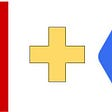## Stop the Stopwords using Different Python Libraries## Neural Machine Translation using word level seq2seq model## Deep Neural Networks Tutorial with TensorFlow## Birdwatching with Convolutional Neural Networks## Recall and Precision## Understanding Deep Learning requires rethinking Generalization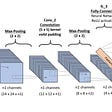## Learning Machine Learning — Part 1: Linear Regression, Cost Functions and Gradient Descent## Tuan Nguyen

PhD student, Senior Software Developer

## Monitoring errors in your A/B tests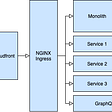## Airflow ETL With EKS EFS & Sagemaker